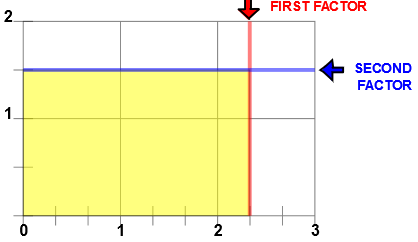# Multiply with Number Line Models

## Learn or teach by designing your own multiply fraction examples. All examples are modeled with a rectangular array.

MULTIPLY WITH NUMBER LINE MODELS
SHOW COLOR
EXPLAIN
SHOW INPUT

# INSTRUCTIONS

Multiply with Number Line Models uses Number lines to demonstrate multiplication of fractions.

You can input the two factors for a multiplication of fractions example. The first factor must be less than 6 and the second factor must be less than 4.

If you want just a whole number for the first or second factor, type in 0 (zero) for a numerator. If you do not want a whole number, type in 0 (zero) for the whole number. Do not type in 0 for the denominator.

With this program you can demonstrate a mixed number times a mixed number.

Uncheck the <EXPLAIN> check box to turn off the answer and the explanation. You can ask your students to complete the number sentence.

Uncheck the <SHOW INPUT> check box to make the input dialog boxes work like a password input boxes, hiding the numbers you input. With <EXPLAIN> and <SHOW INPUT> unchecked you can ask your students to write a number sentence that explains the picture.

With <EXPLAIN> and <SHOW COLOR> unchecked you can ask your students to complete the picture by shading the product and completing a number sentence that explains the picture.

The below image was made by entering 2 13 for the first factor and 1 12 for the second factor. The product is 3 12. You can see in the picture two whole units, two 12 units, a one-third unit and a 1/6 unit. :Added together you have 1+1 + 13 + 12 + 12 + 16 or 3 12 units.See Some Number System Properties for more suggestions for using this page to show some important math principles.

WINDOWS COMPUTERS

Windows users can select any part of the screen by right clicking and selecting "Take a screenshot". Adjust to fit mage you want. This copies the selection into Windows Clipboard™. The screen can then be pasted into Windows Paint™ or your favorite imaging program. Or you can select "Download" which will put the image into your files "Download" folder.